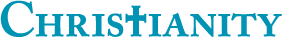## Voting Comparisons arranged by Day of the Week

0

(click on this box to dismiss)Q&A for committed Christians, experts in Christianity and those interested in learning more

``````-- Voting Comparisons arranged by Day of the Week

SELECT
CASE WHEN PostTypeId = 1 THEN 'Question' ELSE 'Answer' END As [Post Type],
DATENAME(WEEKDAY, p.CreationDate) AS Day,
Count(*) AS [Post Count],
SUM(CASE WHEN VoteTypeId = 2 THEN 1 ELSE 0 END) AS [Up Votes],
SUM(CASE WHEN VoteTypeId = 3 THEN 1 ELSE 0 END) AS [Down Votes],
ROUND((CAST((SUM(CASE WHEN VoteTypeId = 2 THEN 1 ELSE 0 END) + SUM(CASE WHEN VoteTypeId = 3 THEN 1 ELSE 0 END)) AS float)) / COUNT(p.Id), 4) AS [Votes per Post],
ROUND((CAST(SUM(CASE WHEN VoteTypeId = 2 THEN 1 ELSE 0 END) AS float) / CAST(SUM(CASE WHEN VoteTypeId = 3 THEN 1 ELSE 0 END) AS float)), 4) AS [Up vs Down Ratio]

FROM
Votes v JOIN Posts p ON v.PostId=p.Id
GROUP BY
PostTypeId, DATEPART(WEEKDAY, p.CreationDate), DATENAME(WEEKDAY, p.CreationDate)
ORDER BY
DATEPART(WEEKDAY, p.CreationDate), PostTypeId``````

### Enter Parameters

Options:
-Hold tight while we fetch your results
:records returned in :time ms:cached